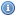# Statistics Books, Documents, and Lectures

 Title Year DescriptionAn Introduction To Probability And Random Processes 1979The purpose of this course is to learn to think probabilistically. The authors start off with a bird's-eye view of probability, then they introduce the theorems, and by the end of the course the student should be able to think probabilistically.Concepts and Applications of Inferential StatisticsThese pages form a free, full-length, and occasionally interactive statistics textbook.Introduction to Probability 2006This text is designed for an introductory probability course taken by sophomores, juniors, and seniors in mathematics, the physical and social sciences, engineering, and computer science.Lectures on Statistics (Robert Ash, University of Illinois at Urbana) 2007These lectures cover a first course in mathematical statistics. Basic probability is a prerequisite, but no prior knowledge of statistics is assumed.Using R for Data Analysis and Graphics 2008Notes designed to allow individuals who have a basic grounding in statistical methodology to work through examples that demonstrate the use of R.